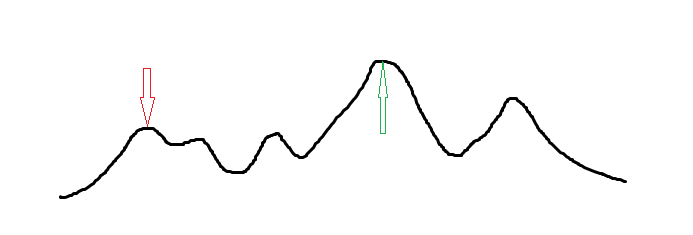# 爬山算法

## 实现¶## 代码¶

  1 2 3 4 5 6 7 8 9 10 11 12 13 14 15 16 17 18 19 20 21 22 23 24 25 26 27 28 29 30 31 32 33 #include #include const int N = 10005; int n, x[N], y[N], w[N]; double ansx, ansy; void hillclimb() { double t = 1000; while (t > 1e-8) { double nowx = 0, nowy = 0; for (int i = 1; i <= n; ++i) { double dx = x[i] - ansx, dy = y[i] - ansy; double dis = sqrt(dx * dx + dy * dy); nowx += (x[i] - ansx) * w[i] / dis; nowy += (y[i] - ansy) * w[i] / dis; } ansx += nowx * t, ansy += nowy * t; if (t > 0.5) t *= 0.5; else t *= 0.97; } } int main() { scanf("%d", &n); for (int i = 1; i <= n; ++i) { scanf("%d%d%d", &x[i], &y[i], &w[i]); ansx += x[i], ansy += y[i]; } ansx /= n, ansy /= n; hillclimb(); printf("%.3lf %.3lf\n", ansx, ansy); return 0; }